A procedure for determining the behavior of anth order Ordinary Differential Equation at a Removable Singularity without actually solving the equation. Consider(1)

where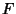is Analytic inand rational in its other arguments. Proceed by making the substitution(2)

with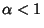. For example, in the equation(3)

making the substitution gives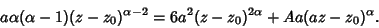(4)

The most singular terms (those with the most Negative exponents) are called the dominant balance terms,'' and must balance exponents and Coefficients at the Singularity. Here, the first two terms are dominant, so(5)(6)

and the solution behaves as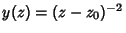. The behavior in the Neighborhood of the Singularity is given by expansion in a Laurent Series, in this case,(7)

Plugging this series in yields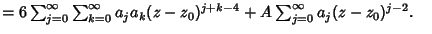(8)
This gives Recurrence Relations, in this case with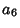arbitrary, so theterm is called the resonance or Kovalevskaya Exponent. At the resonances, the Coefficient will always be arbitrary. If no resonance term is present, the Pole present is not ordinary, and the solution must be investigated using a Psi Function.